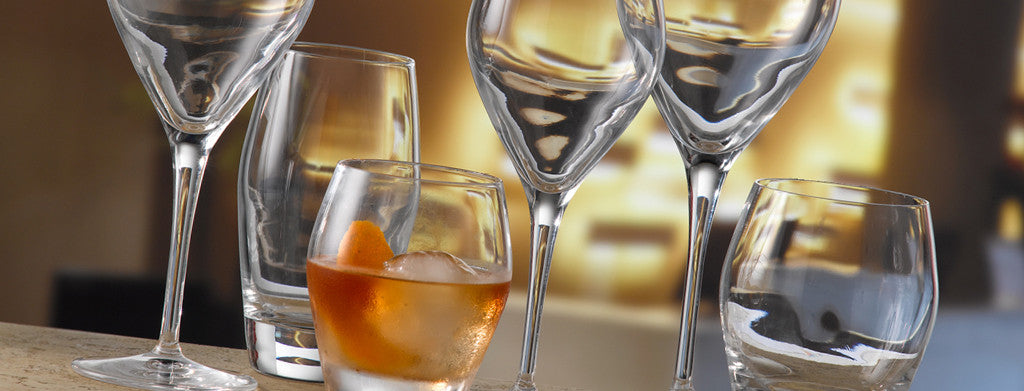Shopping Cart

### Refine

View all

#### Price# Glassware£1.94 each (ex VAT)£3.87 each (ex VAT)£1.84 each (ex VAT)£6.79 each (ex VAT)£5.96 each (ex VAT)£4.24 each (ex VAT)£7.41 each (ex VAT)£5.36 each (ex VAT)£4.75 each (ex VAT)£5.26 each (ex VAT)£4.43 each (ex VAT)£6.16 each (ex VAT)£3.91 each (ex VAT)£3.95 each (ex VAT)£3.34 each (ex VAT)£4.00 each (ex VAT)£7.70 each (ex VAT)£59.00 each (ex VAT)£2.38 each (ex VAT)£4.24 each (ex VAT)£6.40 each (ex VAT)£7.17 each (ex VAT)£5.96 each (ex VAT)£4.97 each (ex VAT)£7.36 each (ex VAT)£4.23 each (ex VAT)£2.37 each (ex VAT)£1.44 each (ex VAT)£1.75 each (ex VAT)£1.16 each (ex VAT)£1.55 each (ex VAT)£2.09 each (ex VAT)£2.07 each (ex VAT)£2.49 each (ex VAT)£1.90 each (ex VAT)£1.85 each (ex VAT)£4.91 each (ex VAT)£1.46 each (ex VAT)£1.64 each (ex VAT)£1.02 each (ex VAT)£1.49 each (ex VAT)£2.30 each (ex VAT)£1.93 each (ex VAT)£2.24 each (ex VAT)£0.97 each (ex VAT)£0.81 each (ex VAT)£0.76 each (ex VAT)£1.29 each (ex VAT)